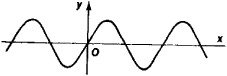# Sinusoid

(redirected from Sinusoids)
Also found in: Dictionary, Thesaurus, Medical.

## sinusoid

[′sī·nə‚sȯid]
(anatomy)
Any of the relatively large spaces comprising part of the venous circulation in certain organs, such as the liver.
(mathematics)

## Sinusoid

(or sine curve), the graph of the function y = sin x. It is a plane curve (see Figure 1) representing the variation in the sine as the argument, or angle, changes. A sinusoid intersects the x-axis at the points 180°k (or πk) and has maxima at the points 90° + 360°k (or π/2 + 2-πk) and minima at the points - 90° + 360°k (or - π/2 + 2πk), where k = 0, ± 1.....Figure 1

The curve defined by the equation y = A sin (ωx + Φ0) is also sometimes called a sinusoid. The relation between this curve and the curve y = sin x is as follows: first, the x-coordinates of the points of y = sin x are increased by a factor of ω; second, the y-coordinates of the points of y = sin x are increased by a factor of A; and third, the curve is translated by an amount - Φ0/ω along the x-axis. The number A is called the amplitude, ω is called the angular frequency, and Φ/0 is called the initial phase angle. The sinusoid is of great importance in the theory of oscillations. [23–1296–]

References in periodicals archive ?
Moreover, the red pulp of splenic tissues showed significant congestion of blood vessels and sinusoids with mild edematous changes (Fig.
Hepatic Stellate Cell (HSC): Cell type found in the liver with several long protrusions that wrap around the sinusoids.
In the present study liver histological sections stained with HandE from rats receiving APAP showed multiple large foci of degenerated hepatocytes with vacuolated cytoplasm and pyknotic nuclei in addition to congestion of hepatic blood sinusoids.
Fine structure of hepatic sinusoids and their development in human embryos and fetuses.
The sinusoids were dilated as compared to control animals, with congestion.
DCTs express a function or a signal in terms of a sum of sinusoids with different frequencies and amplitudes.
Upon selection of STFT, the next step is to select an appropriate window size as , where two closest sinusoids can be distinguished using (7).
Gaertner's 1904 catalog advertised a machine that could add up to 20 sinusoids and a larger version that calculated with 80 sinusoids.
Liver resident macrophages termed Kupffer cells (KCs) are ideally located within the blood stream of the liver sinusoids to encounter intestinal antigens.

Site: Follow: Share:
Open / Close